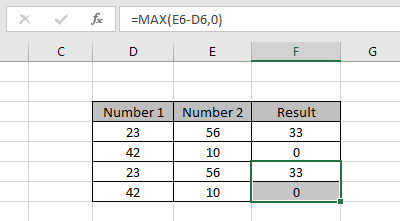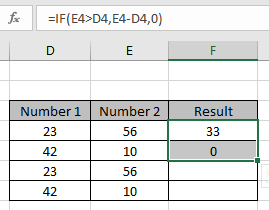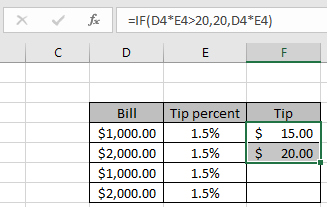# Use MAX/MIN function to replace long IF Excel formulaIn this article, we will learn how to use the MAX/MIN function to replace the long IF Excel formula.

In simple words, while working with numbers, sometimes we need to extract numbers on the basis of given condition. Example if we need to apply the subtraction operator on numbers and don't require negative numbers as result, So force the result to zero instead of negative number. operation inside the formula executed using the operators.

### Avoid Negative Numbers Using MAX

Generally, we use the IF function to avoid negative numbers. Using IF function we need to give three arguments to the function to return the result of applied operation.
Formula :

= IF ( num 1 > num 2, num 1 - num 2, zero )

But here we explained the replacement of the IF function using the MAX function.
Formula :

= MAX ( num 1 - num 2, zero )

Example:
All of these might be confusing to understand. So, let's test this formula via running it on the example shown below. Here we have some data to compute the above explained process.As you can see from the above two values, we used IF function to extract criteria results. Now we can do the exact same operation using the MAX function as shown below.As you can see the MAX function takes one argument with subtract operation and other is zero and the formula returns whichever is greater.

### Capping Using the MIN Function

Let's say you want to multiply two numbers. If the result exceeds a limit, it should print the limit or print the result. Generally, we would go for an IF formula to do this but MIN function can do it faster and elegantly.
Formula :

= IF (  num1 *num2>limit,limit, num1* num2)

But here we explained the replacement of IF function using the MIN function.

Formula :

= MIN ( num1* num2, min )

Example:

All of these might be confusing to understand. So, let's test this formula via running it on the example shown below. Here we have a calculation data to compute the above explained process. We need to pay the tip ( 1.5% of total bill ) to the waiter and the max amount you can pay is \$ 20.00. See the example below.As you can see from the above two values, we used the IF function to extract tip results. Now we can do the exact same operation using the MIN function as shown below.As you can see the MIN function takes one argument with percent operation and the other is the max amount where the formula returns whichever is smaller.

Here are some observational notes shown below.

Notes:

1. The formula only works with numbers only.
2. Logic Operators like equals to ( = ), less than equal to ( <= ), greater than ( > ) or not equals to ( <> ) can be performed within function applies with number only.
3. Mathematical operators like SUM ( + ), Subtract ( - ), product ( * ), division ( / ), percent ( % ), power ( ^ ) can be performed over numbers using the example.

Hope this article about how to use the MAX/MIN function to replace long IF Excel formula is explanatory. Find more articles on COUNTIFS functions here.

If you liked our blogs, share it with your friends on Facebook. And also you can follow us on Twitter and Facebook. We would love to hear from you, do let us know how we can improve, complement or innovate our work and make it better for you. Write to us at info@exceltip.com

Related Articles

IF with AND function : Implementation of logic IF function with AND function to extract results having criteria in Excel.

IF with OR function : Implementation of logic IF function with OR function to extract results having criteria in excel data.

How to use nested IF function : nested IF function operates on data having multiple criteria. The use of repeated IF function is nested IF excel formula.

SUMIFS using AND-OR logic : Get the sum of numbers having multiple criteria applied using logic AND-OR excel function.

Minimum value using IF function : Get the minimum value using the excel IF function and MIN function on array data.

How to use wildcards in excel : Count cells matching phrases using the wildcards in excel

Popular Articles

50 Excel Shortcut to Increase Your Productivity : Get faster at your task. These 50 shortcuts will make you work even faster on Excel.

The VLOOKUP Function in Excel : This is one of the most used and popular functions of excel that is used to lookup value from different ranges and sheets.

COUNTIF in Excel 2016 : Count values with conditions using this amazing function. You don't need to filter your data to count specific values. Countif function is essential to prepare your dashboard.

How to Use SUMIF Function in Excel : This is another dashboard essential function. This helps you sum up values on specific conditions.

Terms and Conditions of use

The applications/code on this site are distributed as is and without warranties or liability. In no event shall the owner of the copyrights, or the authors of the applications/code be liable for any loss of profit, any problems or any damage resulting from the use or evaluation of the applications/code.